# Creating a Pandas Series

• Difficulty Level : Basic
• Last Updated : 22 Jun, 2022

Pandas Series is a one-dimensional labeled array capable of holding data of any type (integer, string, float, python objects, etc.). The axis labels are collectively called index. Labels need not be unique but must be a hashable type. The object supports both integer and label-based indexing and provides a host of methods for performing operations involving the index.To create Series with any of the methods make sure to import pandas library.

Creating an empty Series: Series() function of Pandas is used to create a series. A basic series, which can be created is an Empty Series.

## Python3

 `# import pandas as pd` `import` `pandas as pd`   `# Creating empty series` `ser ``=` `pd.Series()`   `print``(ser)`

Output :

`Series([], dtype: float64)`

By default, the data type of Series is float.

Creating a series from array: In order to create a series from NumPy array, we have to import numpy module and have to use array() function.

## Python3

 `# import pandas as pd` `import` `pandas as pd`   `# import numpy as np` `import` `numpy as np`   `# simple array` `data ``=` `np.array([``'g'``, ``'e'``, ``'e'``, ``'k'``, ``'s'``])`   `ser ``=` `pd.Series(data)` `print``(ser)`

Output: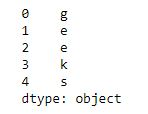By default, the index of the series starts from 0 till the length of series -1.

Creating a series from array with an index: In order to create a series by explicitly proving index instead of the default, we have to provide a list of elements to the index parameter with the same number of elements as it is an array.

## Python3

 `# import pandas as pd` `import` `pandas as pd`   `# import numpy as np` `import` `numpy as np`   `# simple array` `data ``=` `np.array([``'g'``, ``'e'``, ``'e'``, ``'k'``, ``'s'``])`   `# providing an index` `ser ``=` `pd.Series(data, index``=``[``10``, ``11``, ``12``, ``13``, ``14``])` `print``(ser)`

Output: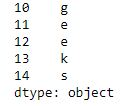Creating a series from Lists: In order to create a series from list, we have to first create a list after that we can create a series from list.

## Python3

 `import` `pandas as pd`   `# a simple list` `list` `=` `[``'g'``, ``'e'``, ``'e'``, ``'k'``, ``'s'``]`   `# create series form a list` `ser ``=` `pd.Series(``list``)` `print``(ser)`

Output :Creating a series from Dictionary: In order to create a series from the dictionary, we have to first create a dictionary after that we can make a series using dictionary. Dictionary keys are used to construct indexes of Series.

## Python3

 `import` `pandas as pd`   `# a simple dictionary` `dict` `=` `{``'Geeks'``: ``10``,` `        ``'for'``: ``20``,` `        ``'geeks'``: ``30``}`   `# create series from dictionary` `ser ``=` `pd.Series(``dict``)`   `print``(ser)`

Output: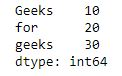Creating a series from Scalar value: In order to create a series from scalar value, an index must be provided. The scalar value will be repeated to match the length of the index.

## Python3

 `import` `pandas as pd`   `import` `numpy as np`   `# giving a scalar value with index` `ser ``=` `pd.Series(``10``, index``=``[``0``, ``1``, ``2``, ``3``, ``4``, ``5``])`   `print``(ser)`

Output: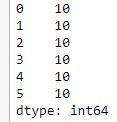Creating a series using NumPy functions : In order to create a series using numpy function, we can use different function of numpy like numpy.linspace(), numpy.random.radn()

## Python3

 `# import pandas and numpy` `import` `pandas as pd` `import` `numpy as np`   `# series with numpy linspace()` `ser1 ``=` `pd.Series(np.linspace(``3``, ``33``, ``3``))` `print``(ser1)`   `# series with numpy linspace()` `ser2 ``=` `pd.Series(np.linspace(``1``, ``100``, ``10``))` `print``(& quot` `       ``\n"       , ser2)`

Output: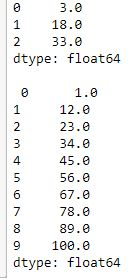Creating a Series using range function:

## Python3

 `# code` `import` `pandas as pd` `ser``=``pd.Series(``range``(``10``))` `print``(ser)`

```Output:
0    0
1    1
2    2
3    3
4    4
5    5
6    6
7    7
8    8
9    9
dtype: int64```

Creating a Series using for loop and list comprehension:

## Python3

 `import` `pandas as pd` `ser``=``pd.Series(``range``(``1``,``20``,``3``), index``=``[x ``for` `x ``in` `'abcdefg'``)]` `print``(ser)`

Output:

```a     1
b     4
c     7
d    10
e    13
f    16
g    19
dtype: int64```

Creating a Series using mathematical expressions:

## Python3

 `import` `pandas as pd` `import` `numpy as np` `ser``=``np.arange(``10``,``15``)` `serobj``=``pd.Series(data``=``ser``*``5``,index``=``ser)` `print``(serobj)`

Output:

```10    50
11    55
12    60
13    65
14    70
dtype: int32```

My Personal Notes arrow_drop_up
Recommended Articles
Page :